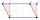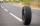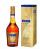# Unit conversion + expression of a variable from the formula - math problems

#### Number of problems found: 90

• RainIt rains at night. On 1 m2 of lake will drop 60 liters of water. How many cm will the lake level rise?
• The parallelogramThe parallelogram has one side 2 dm long, which is one-sixth of its circumference. How many cm does the other side of the parallelogram measure?
• A clockA clock was set right at 6:00 AM. If it gains 3 1/2 minutes per hour, what time will it show at 6:00 PM on the same day? Show your solution
• Bike wheelThe bike wheel has a radius of 30cm. How many times does it turn if we go on a 471m bike?
• Thousand ballsWe have to create a thousand balls from a sphere with a diameter of 1 m. What will be their radius?
• DecibelBy what percentage does the sound intensity increase if the sound intensity level increases by 1 dB?
• The potThe pot is a cylinder with a volume of V = 7l and an inner diameter of d = 20cm. Find its depth.
• PotCalculate the height of 3 liter pot with shape cylinder with a diameter of 10 cm.
• Cylinder containerThe cylindrical container with a diameter of 1.8 m contains 2,000 liters of water. How high does the water reach?
• DecagonCalculate the area and circumference of the regular decagon when its radius of a circle circumscribing is R = 1m
• Cylindrical tankCylindrical tank holds 600hl water and is deep 2.5 m. Calculate the diameter of the cylinder.
• The tankThe tank is full up to 4/5 of the total height and contains 240 hl of water. The area of the base is 6 square meters. What is the height of the tank?
• BottleA company wants to produce a bottle whose capacity is 1.25 liters. Find the dimensions of a cylinder that will be required to produce this 1.25litres if the hight of the cylinder must be 5 times the radius.
• AlcoholHow many cubic centimeters of 96% alcohol is needed to obtain 0.75 liter of 40% alcohol?
• Tank diameterA cylindrical tank has a volume of 60 hectoliters and is 2.5 meters deep. Calculate the tank diameter.
• Water tank300hl of water was filled into the tank 12 m long and 6 m wide. How high does it reach?
• Hectoliters of waterThere are 942 hectoliters of water in a cylindrical tank with an inner diameter of 6 m. The water reaches two thirds of the depth of the tank. Calculate its depth.
• Cylinder heightCalculate the height of the cylinder and its surface is 2500 dm2 and the bases have a diameter 5dm.
• Aquarium heightHow high does the water in the aquarium reach, if there are 36 liters of water in it? The length of the aquarium is 60 cm and the width is 4 dm.
• ContainerThe container has a cylindrical shape the base diameter 0.8 m and the area of the base is equal to the area of the wall. How many liters of water can we pour into the container?

Do you have an interesting mathematical word problem that you can't solve it? Submit a math problem, and we can try to solve it.

We will send a solution to your e-mail address. Solved examples are also published here. Please enter the e-mail correctly and check whether you don't have a full mailbox.

Please do not submit problems from current active competitions such as Mathematical Olympiad, correspondence seminars etc...

Unit conversion - math word problems. Expression of a variable from the formula - math word problems.